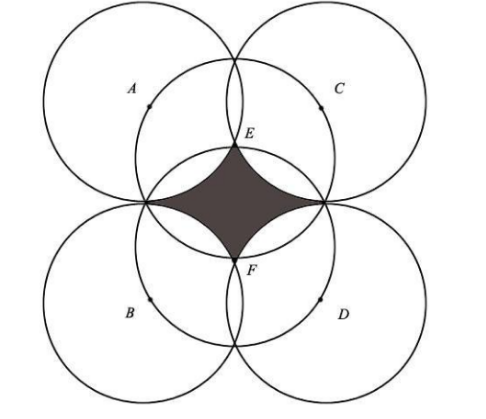# Circle funIf all circles with centers $A, B, C, D, E$ and $F$ have the same radius of 7, what is the area of the shaded region given that circles $E$ and $F$ pass through the centers of the other circles and that ABCD forms a rectangle (when joining up those points with lines).

Give answers to at least one decimal place.​

×